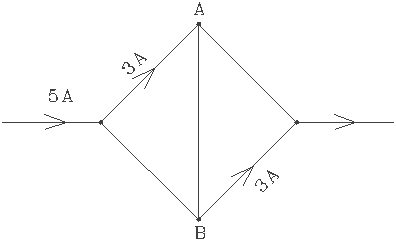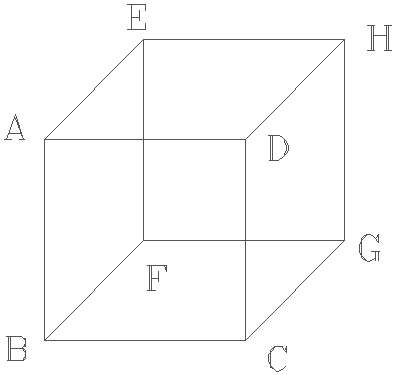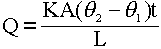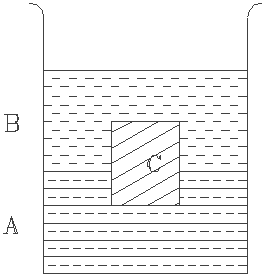SCHOLASTIC APTITUDE TEST 1995.

PHYSICS

 Time: ONE Hour Max. Marks: 60

• Answers must be written either in English or the medium of instruction of the candidate in high school.
• There will be no negative marking.
• There are FOUR Sections. Answer all questions of a section at one place.
• Use of calculators or graph papers is not permitted.
• Marks alloted for individual sections are as follows. All questions in an individual section carry equal marks.
 Section I 10 Marks Section II 20 Marks Section III 15 Marks Section IV 15 Marks

SECTION I

1. Velocity of light in centimeter per nanosecond is _______________________ .
2. Phase of vibrating particles varies linearly and continuously when a wave travels in a medium. The linear distance between particles differing in phase by p when a wave of frequency 2 kHz travels at 350 m/s is a medium is __________________.
3. Density and youngs modulus of a certain hypothetical material are 5 gm/cm3 and 2.0 x 1010 Pa. Velocity of sound wave in this material is ______________.
4. Field strength at a certain distance from a charge Q is E. Field strength at one fourth of this distance from a charge Q/4 is _______________ .
5. Farad x Ohm expressed in the simplest form equals _________________.
6. A conductor carries a current vertically upwards. Magnetic field due to this current to the East of the conductor would be directed ____________________________.
7. An electron is moving southward in a region of space having a magnetic field directed vertically upwards. It experiences a force towards _________________ .
8. The change that takes place in the nucleus when a beta particle is emitted is ________________.
9. Two Deutrons combine to form a Helium nucleus. The masses of Deuterium and Helium atoms are 2.0141 u and 4.0026 u respectively. The energy yield in MeV per reaction is ________________.
10. Electromagnetic radiations of wavelength 5 nm fall in the _________________ region.

SECTION II

1. A man moves 100 m 30° East of south then another 100 m 60° North of East. He is at a distance of _________________ from the starting point.
2. Displaced air exerts a vertcally upward force equal to its own weight on a body. Force exerted by the displaced air on a man of weight 60 kg is nearly __________________ . ( Average density of a human being is nearly same as that of water and density of air is 1.3 gm/l. ).
3. A mirror produces a magnified image of a linear object placed 30 cm away from it 30 cm away from the object. If the object is moved towards the mirror by 5 cm the image moves by ____________.
4. With velocity of light in vaccum chosen as a standard the refractive indicies of water and glycerine are 4/3 and 8/5 respectively. If velocity of light in water is chosen as standard refractive index of glycereine would be ___________________ .
5. A source of sound of frequency 5 kHz is moving at 20 m/s. The wavelength of waves in front of the source is _______________________ .( Velocity of sound in air is 350 m/s ).
6. Two wires of circular cross section are made of equal amounts of same material and have their radii in the ratio 1:2. The ratio of their electrical resistances is ___________________.
 Current between junctions A and B is _______________ .ABCDEFGH is a wireframe of twelve conductors forming the edges of a cube. Resistance between any two adjacent corners is R. If the connection between A,E and C,G are removed the effective resistance between B and D would be ________________.1. A battery is rated 12 V and has a capacity of 12 Ah. ( 1 Ah means the battery can drive one ampere of current for one hour or half ampere for two hours and so on..... ). The energy it can deliver is _______________________.
2. An electric lamp is rated 40 W at 200 V. If the lamp is operated at 150 V its power would be __________________.

SECTION III

The amount of heat Q flowing along the length of a rod in a time t is given by the expressionWhere q 2 and q 1 are temperatures of hot and cold ends, A and L are area of cross section and length of the rod, and K the coefficient of thermal conductivity ( a constant for the given material ) of the rod. It is assumed that there is no heat loss from the sides of the rod.

1. A rod is 50 cm long and has a cross sectional area of 10 mm2. If coefficient of thermal conductivity of the material of the conductor is 400 W/mK, the rate of heat flow along the rod is _____________________.
2. Temperature gradient is the rate at which the temperature varies with the length of the rod and is a constant for a rod carrying heat in steady state. If the temperature gradient of a rod is 5 K/mm, the heat that flows in one minute through a rod of cross sectional area 2 cm2 and coefficient of thermal conductivity 250 W/mK is equal to
________________.
3. When two rods are placed end to end the rate of heat flow in them must be same. If two rods of equal areas of cross section and having their lengths and coefficients of thermal conductivities in the ratio 1:2 and 2:1 respectively have their free ends maintained at 350 K and 300 K respectively, the temperature of the junction will be _______________.
4. The amount of heat that escapes from a house through a 3 m x 2 m glass window pane of thickness 5 mm for a temperature difference of 10 C° in one hour is ____________________. ( Coefficient of thermal conductivity of glass is 0.008 W/cmK )
5. One end of rod of cross sectional area 5 cm2, length 25 cm, coefficient of thermal conductivity 210 W/mK is maintained at 100° C and the other end is connected to beaker of water containing melting ice. The amount of ice that melts in a minute is _______________. ( Latent heat of fusion of ice is 80 cal/gm )

SECTION IV

A body floats when the weight of the liquid it displaces equals its own weight. Questions in the following section are based on this principle.

1. A block of ice (density 900 kg/m3) is floating in a beaker of liquid (density 1200 kg/m3). The fraction of its volume seen above the liquid is ____________________ .
2. A body of density 1200 kg/m3 just floats in a homogenous mixture of two liquids of densities 1000 kg/m3 and 1500 kg/m3. The ratio by volumes in which the liquids are mixed is ____________________ .
3. A cubical block of Aluminum has a cavity inside. If it floats in water with 7/8 of it’s volume submerged in water. The ratio of the volume of the material to the ratio of its external volume is __________________ .
 A cubical block C floats as shown with one fourth of its volume submerged in liquid A and the remaining three fourths in liquid B as shown. If density of liquid A is 600 kg/m3 and that of B is 400 kg/m3, density of the material of the block is _____________________.1. A test tube containing some lead shot floats vertically in a beaker of water. A few lead shots are removed from the tube and dropped in to the beaker. The level of water in the beaker _______________ .( Assume that the test tube continues to float vertically after the lead shots are removed from it ).

Home